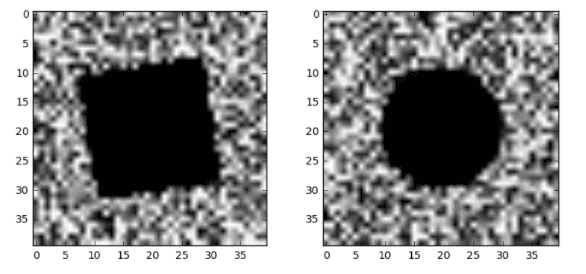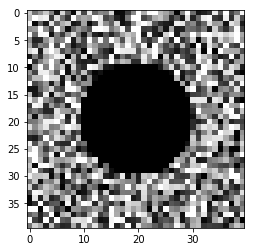#### numpy.array转换为图片并显示出来

统计/机器学习 计算机视觉 数据可视化 Python 数据竞赛    浏览次数：7511        分享

0剪叔   2017-12-29 14:57

##### 2个回答
7

# ipython@python3环境
import pandas as pd
import numpy as np

with open('~/train.csv') as f:

X = data.drop(['y','id'], axis=1)
X = np.array(X)

#  每一行代表一张图片，选取第一行。
pic1 = X
# 图片分辨率是40*40
pic1 = pic1.reshape((40, 40))

# 绘图
%matplotlib inline
from matplotlib import pyplot as plt
# 传入cmap='gray'指定图片为黑白
plt.imshow(pic1, cmap='gray')pic1.reshape((height, width, 3))

SofaSofa数据科学社区DS面试题库 DS面经s3040608090   2017-12-29 15:43

2

import matplotlib.pyplot as plt
plt.imshow(my_figure, cmap=plt.cm.binary)
plt.show()


SofaSofa数据科学社区DS面试题库 DS面经数据痴汉   2018-03-23 01:19相关主题